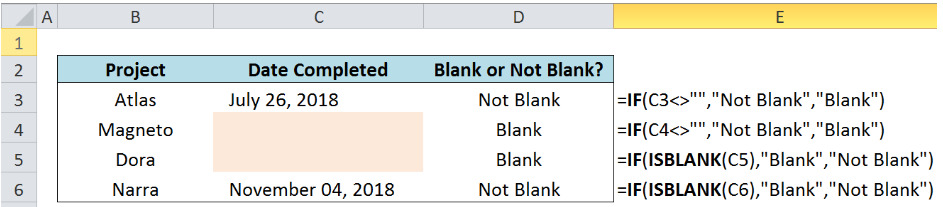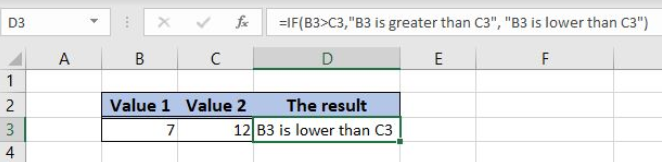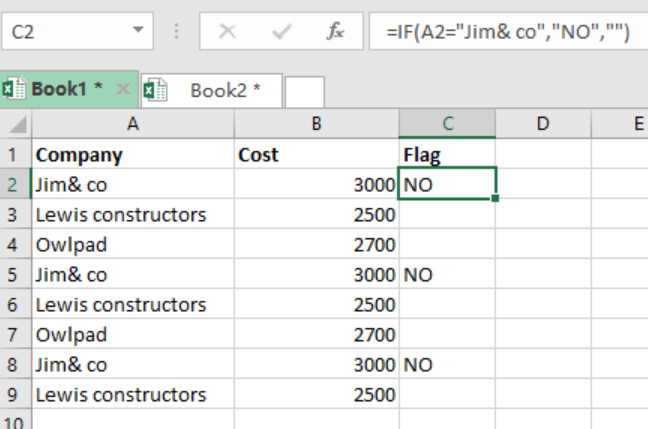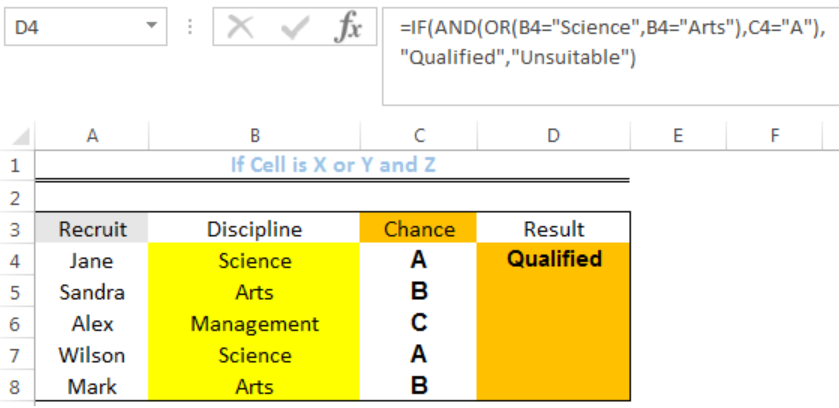Get instant live expert help with Excel or Google Sheets“My Excelchat expert helped me in less than 20 minutes, saving me what would have been 5 hours of work!”

#### Post your problem and you’ll get expert help in seconds.

Your message must be at least 40 characters
Our professional experts are available now. Your privacy is guaranteed.

# Excel IF Function Examples – IF THEN, IF with Multiple Criteria, IF ELSE

Excel’s IF function allows you to perform a logical test on your data and return one value if the result is TRUE and another if it is FALSE. There are a variety of ways to use the IF function, some more complex than others. This is a list of the various tutorials for using the IF function so that you can learn to apply it or troubleshoot issues.

If you can’t find what you need in this list or want to get your issue solved quickly, Excelchat is always available to help.

## Determine if a Cell is Not Blank in Excel

When you need to know whether or not certain cells in your Excel spreadsheet are blank, you can use the IF function. This tutorial explains how to do this using the IF function, the ISBLANK function, and a combination of the two.Determine if a cell is not blank in Excel.

## How to Determine IF a Cell is Blank or Not Blank in Excel

This is another step-by-step guide that shows how to use the IF function to find out if cells in a range are “blank” or “not blank.” The piece teaches you how to create a logical test on a given set of data, with a series of illustrations and examples.

## Learn How to Use Nested IF Statement in Excel

While a logical test with an IF statement can be simple, it can also be more complex, where there are additional formulas within the syntax. This tutorial illustrates how to combine more than one formula, where one uses the IF statement to perform a logical test. It includes illustrations and examples.

## How to Use the IFERROR Function in Excel

Some formulas in Excel return errors. This guide shows how you can use the IFERROR function to tell Excel what to do if an error does occur. It includes the syntax of the IFERROR function as well as several examples of using it with VLOOKUP, INDEX and MATCH, and VLOOKUP with multiple tables.

## IF ELSE

This is a basic guide to using the IF function in Excel. The reader is provided with the generic syntax for the IF function and then given an example, with illustrations, of a logical test using number. For example, “if a number is greater than X = true, if a number is lower than X=false.”Learn how to use the IF function in Excel.

## Force Negative Numbers to Zero

If you have a spreadsheet full of data and you don’t want any negative numbers, this guide shows how to create a formula that will force those numbers to zero. Specifically, it shows how to set up the data and uses the MAX function.

## If Cell Contains This or That

You can check cells in a spreadsheet or a range of data for certain data. This tutorial shows how to see if a range of cells contains more than one selected value (this or that), using the COUNTIF, IF, and SUM functions.

## If Cell Contains

Finding data in a large Excel spreadsheet can be a challenge without the right tools. This guide shows you how to locate data based on a single criterion. In other words, you can see if a cell contains a certain term using the SEARCH, IF, and ISNUMBER functions.

## How to Write an IF Statement in Excel

This is a tutorial on using the IF function in Excel for a logical test. The reader is given the syntax for the IF function, including an explanation of the terms. There are also rules for using this statement and an example with illustrations.

## IF Cell Equals

Find out how to use the Excel IF statement to determine whether a cell equals a certain value with this guide. This piece gives an explanation of the formula as well as a detailed example and illustrations to follow.Learn how to determine IF a cell equals a specific value.

## If This AND That OR That

Even when using the IF statement, you might have several things that you want Excel to consider or do. This piece shows how you can combine the IF function with AND, OR, or both to produce the desired result.

## How to Use the IF and OR Functions in Excel

When you evaluate a dataset, you might need to run several logical tests or test multiple conditions. When there is more than one condition, this tutorial shows how to combine the IF function with the OR function to get the desired result.

## How to Check If a Cell is Greater Than

Do you need to know if the value of a cell is greater than a certain figure or the value of another cell? This step-by-step guide will show you how to use the IF function to figure out if the value of one cell is greater than your criteria.

## How to Use “IF Cell is This or That”

This tutorial illustrates how to figure out if a cell contains one of two criteria using the IF and OR functions. Specifically, it provides the syntax that includes the “this” or “that” operators and gives an example of its use.

## IF Cell is X or Y or Z

In this piece, you will learn how to create a nested formula that will test several conditions. It uses the IF, AND, and OR functions, and there is an example with illustrations to use as a guide.Learn to check IF a cell is X or Y or Z.

## Nested IF Function Example

This is another tutorial piece that illustrates how to set up a nested formula that includes the IF function. The guide explains why this might be used, gives the formula syntax, and provides a detailed example with illustrations.

Did you find what you need on this list? If not, Excelchat has experts standing by 24/7 that can give you instruction on Excel functions or provide problem-specific help. Your first session is always free.